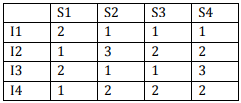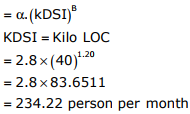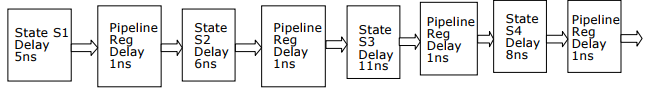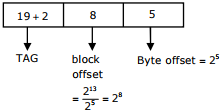# Computer Organization and Architecture - Online Test

Q1. How many 32K × 1 RAM chips are needed to provide a memory capacity of 256 K-bytes =
Explaination / Solution:
No Explaination.

Q2. Consider a 4 stage pipeline processor. The number of cycle needed by the four instructions I1,I2,I3,I4 in stages S1, S2, S3, S4 is shown below:What is the number of cycles needed to execute the following loop? for (i=1 to 2) {I1; I2; I3; I4 ;}
Explaination / Solution:
No Explaination.

Q3. A multilevel page table is preferred in comparison to a single level page table for translating virtual address to physical address because
Explaination / Solution:
No Explaination.

Q4. Consider a hypothetical processor with an instruction of type LW R1, 20(R2), which during execution reads a 32-bit word from memory and stores it in a 32-bit register R1. The effective address of the memory location is obtained by the addition of constant 20 and the contents of register R2. Which of the following best reflects the addressing mode implemented by this instruction for the operand in memory?
Explaination / Solution:

Here 20 will act as base and content of R2 will be index

Q5. A company needs to develop digital signal processing software for one of its newest inventions. The software is expected to have 40000 lines of code. The company needs to determine the effort in person-months needed to develop this software using the basic COCOMO model. The multiplicative factor for this model is given as 2.8 for the software development on embedded systems, while the exponentiation factor is given as 1.20. What is the estimated effort in personmonths?
Explaination / Solution:

Effort person per monthQ6. Which of the following is NOT desired in a good Software Requirement Specifications (SRS) document?
Explaination / Solution:
No Explaination.

Q7. Consider an instruction pipeline with four stages (S1, S2, S3 and S4) each with combinational circuit only. The pipeline registers are required between each stage and at the end of the last stage. Delays for the stages and for the pipeline registers are as given in the figure.What is the approximate speed up of the pipeline in steady state under ideal conditions when compared to the corresponding non-pipeline implementation?
Explaination / Solution:

(5 + 6 + 11 + 8) / (11 + 1) = 30 / 12 = 2.5

Q8. An 8KB direct mapped write-back cache is organized as multiple blocks, each of size 32-bytes. The processor generates 32-bit addresses. The cache controller maintains the tag information for each cache block comprising of the following. 1 Valid bit 1 Modified bit As many bits as the minimum needed to identify the memory block mapped in the cache. What is the total size of memory needed at the cache controller to store metadata (tags) for the cache?
Explaination / Solution:Required answer =256 × (19 + 2) = 5376 bits

Q9. On a non-pipelined sequential processor, a program segment, which is a part of the interrupt service routine, is given to transfer 500 bytes from an I/O device to memory.
Initialize the count to 500
LOOP: Load a byte from device
Decrement the count
If count != 0 go to LOOP
Assume that each statement in this program is equivalent to a machine instruction which takes one clock cycle to execute if it is a non-load/store instruction. The load-store instructions take two clock cycles to execute.
The designer of the system also has an alternate approach of using the DMA controller to implement the same transfer. The DMA controller requires 20 clock cycles for initialization and other overheads. Each DMA transfer cycle takes two clock cycles to transfer one byte of data from the device to the memory.
What is the approximate speedup when the DMA controller based design is used in place of the interrupt driven program based input-output?
Explaination / Solution:

No. of clock cycles required by using load-store approach = 2 + 500 × 7 = 3502 and that of by using DMA = 20 + 500 × 2 = 1020 Required speed up = 3502/1020 = 3.4

Q10. Register renaming is done is pipelined processors
Explaination / Solution:

Register renaming is done to eliminate WAR/WAW hazards.